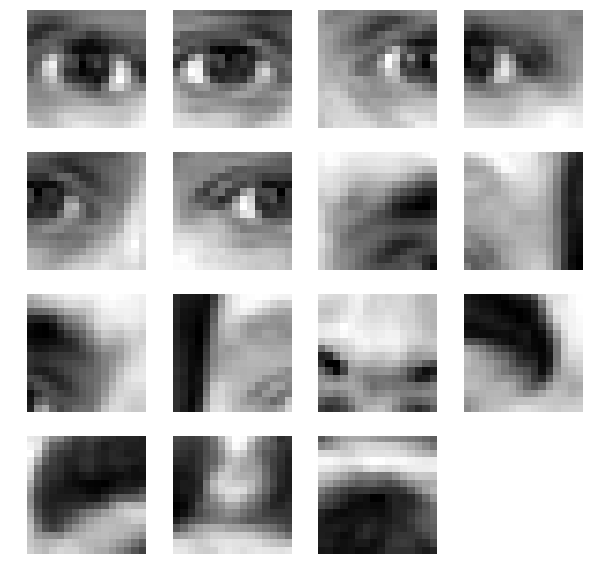# How to (quickly) extract bilinear-interpolated patches from a 2d image at specific points?

I am trying to train a deep learning model to predict face landmarks following this paper. I need to crop parts of the image that contains face into smaller patches around facial landmarks. For example, if we have the image shown below:The function should generate `N=15` “patches”, one patch per landmark:I have the following naïve implementation build on top of `torch` tensors:

``````def generate_patch(x, y, w, h, image):
c = image.size(0)
patch = torch.zeros((c, h, w), dtype=image.dtype)
for q in range(h):
for p in range(w):
yq = y + q - (h - 1)/2
xp = x + p - (w - 1)/2
xd = 1 - (xp - math.floor(xp))
xu = 1 - (math.ceil(xp) - xp)
yd = 1 - (yq - math.floor(yq))
yu = 1 - (math.ceil(yq) - yq)
for idx in range(c):
patch[idx, q, p] = (
image[idx, math.floor(yq), math.floor(xp)]*yd*xd +
image[idx, math.floor(yq),  math.ceil(xp)]*yd*xu +
image[idx,  math.ceil(yq), math.floor(xp)]*yu*xd +
image[idx,  math.ceil(yq),  math.ceil(xp)]*yu*xu
).item()
return patch

def generate_patches(image, points, n=None, sz=31):
if n is None:
n = len(points)//2
patches = []
for i in range(n):
x_val, y_val = points[i], points[i + n]
patch = generate_patch(x_val, y_val, sz, sz, image)
patches.append(patch)
return patches
``````

The code does its work but too slowly. I guess because of all these for-loops and separate pixels indexing. I would like to vectorize this code, or maybe find some C-based implementation that could do it faster.

I know there is the `extract_patches_2d` function from `sklearn` package that helps to pick random patches from the image. However, I would like to pick the patches from specific points instead of doing it randomly. I guess that I can somehow adapt the aforementioned function, or convert the implementation shown above into Cython/C code but probably someone has already done something like this before.

Could you please advise some alternative to the code shown above, or maybe a proposal on how to make it faster? (Except using several parallel workers).

The question was originally posted on the StackOverflow but I am duplicating it here because think it could be interesting to other developers who try to do something similar, and also there are a lot of skilled programmers there.

2 Likes

I guess the original formulation of this question is a bit unclear. To clarify, I am not just cropping the image but applying the bilinear interpolation during the patches extraction process. That’s why the algorithm is more involved than just taking slices.